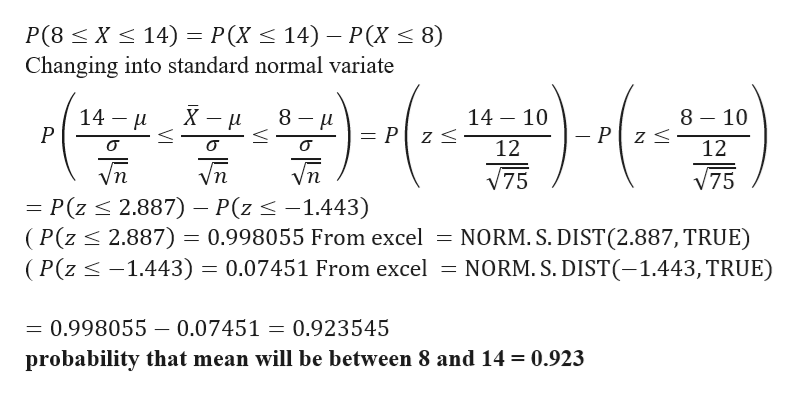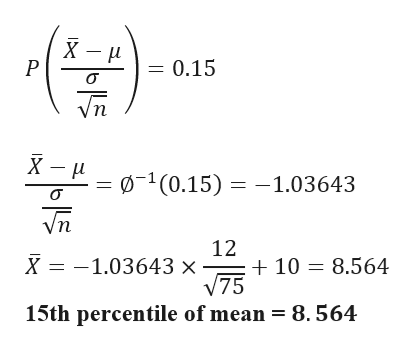# 1. Asample of size 75 will be drawn  from a population with mean 10 and standard deviation 12.a. Find the probability that mean will be between 8 and 14.b. Find the 15th percentile of mean.2. A sample of size 20 will be drawn from a population with mean 6 and standard deviation 3.a. Is it appropriate to use the normal distribution to find probabilities for mean?b. If Appropriate find the probability that mean will be greater than 4.c. If appropriate find the 30th percentile of mean.

Question
34 views

1. Asample of size 75 will be drawn  from a population with mean 10 and standard deviation 12.

a. Find the probability that mean will be between 8 and 14.

b. Find the 15th percentile of mean.

2. A sample of size 20 will be drawn from a population with mean 6 and standard deviation 3.

a. Is it appropriate to use the normal distribution to find probabilities for mean?

b. If Appropriate find the probability that mean will be greater than 4.

c. If appropriate find the 30th percentile of mean.

check_circle

Step 1

Hello there! there are more than one question. According to our policies we cannot answer more than one question. So please find the solution for first question. If you have doubts in remaining  question kindly make a new request, mentioning the question in which you need help.

Given Data

n = 75

mean =10

standard deviation =12

Step 2

a) probability that mean will be between 8 and 14help_outlineImage TranscriptioncloseP(8 X 14) = Changing into standard normal variate Р(X < 14) — Р(X < 8) 8 10 14 10 14 P 8 H _ - Pz = P z < о 12 12 Vn /75 /75 Р(2 < 2.887) — P(z <-1.443) (P(z 2.887) = 0.998055 From excel (P(z <-1.443) = 0.07451 From excel NORM. S. DIST (2.887, TRUE) NORM. S. DIST(-1.443, TRUE) 0.998055 0.07451 0.923545 probability that mean will be between 8 and 14 = 0.923 fullscreen
Step 3

b) 15th percentil...help_outlineImage Transcriptionclose= 0.15 P (0.15) 1.03643 12 10 8.564 75 X 1.03643 x 15th percentile of mean = 8.564 fullscreen

### Want to see the full answer?

See Solution

#### Want to see this answer and more?

Solutions are written by subject experts who are available 24/7. Questions are typically answered within 1 hour.*

See Solution
*Response times may vary by subject and question.
Tagged in

### Other